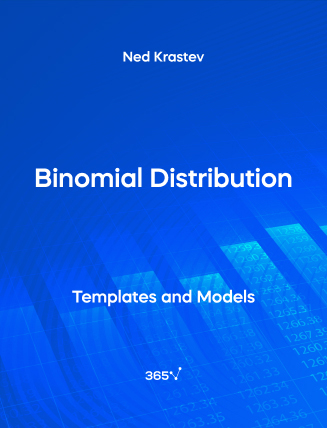## Binomial Distribution – Excel Template###### Ned Krastev

Binomial events represent a sequence of identical Bernoulli events. We use the letter “B” to express a Binomial distribution, followed by the number of trials and the probability of success in each one.

Suppose that you bought a single stock of General Motors. Historically, there is a 60% chance that the price of your stock will go up on any given day (that’s when the closing price is higher than the opening price), and a 40% chance it will drop.

With the probability distribution function, we can calculate the likelihood of the stock price increasing 3 times during the 5-work-day week. If we wish to use the probability distribution formula, we need to plug in 3 for “y”, 5 for “n”, and 0.6 for “p”.

Other related topics you might be interested to explore are Normal distribution and Uniform distribution.

This is an open-access Excel template in XLSX format that will be useful to anyone who wants to work as a Statistician, Business Intelligence Analyst, Data Scientist, Portfolio Manager, or Risk Manager.